Next: Lunar Parallax Up: The Moon Previous: Example Longitude Calculations

# Determination of Ecliptic Latitude

A model of the moon's ecliptic latitude is needed in order to predict the occurrence of solar and lunar eclipses. Figure 23 shows the moon's orbit about the earth. The plane of this orbit is fixed, but slightly tilted with respect to the plane of the ecliptic (i.e., the plane of the sun's apparent orbit about the earth). Let the two planes intersect along the line of nodes,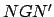. Here,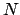is the point at which the orbit crosses the ecliptic plane from south to north (in the direction of the moon's orbital motion), and is termed the ascending node. Likewise,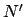is the point at which the orbit crosses the ecliptic plane from north to south, and is called the descending node. Incidentally, the line of nodes must pass through point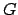, since the earth is common to the ecliptic plane and the plane of the lunar orbit. The angle,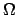, subtended between the radius vector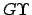, connecting the earth to the vernal equinox, and the line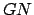, is known as the longitude of the ascending node. Note, incidentally, that the ascending node precessess in the opposite direction to the moon's orbital motion at the rate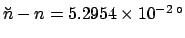per day, orin 18.6 years. This unusually large precession rate is another consequence of the sun's strong perturbing influence on the moon's orbit. Let the line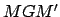lie in the plane of the moon's orbit such that it is perpendicular to. The inclination,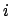, of the moon's orbital plane is the angle that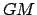subtends with its projection onto the ecliptic plane. Likewise, the moon's ecliptic longitude,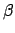, is the angle that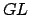subtends with its projection onto the ecliptic plane. Simple geometry yields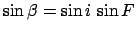, where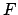is the angle betweenand. This angle is termed the argument of latitude. Now, it is easily seen that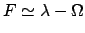, where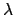is the moon's ecliptic longitude (i.e., the angle subtended betweenand). Here, we are assuming that the orbital inclinationis relatively small. The mean argument of latitude is defined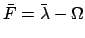. Hence, our model for the moon's ecliptic latitude becomes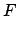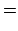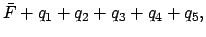(124)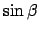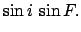(125)

The value of the lunar orbital inclination,, for the J2000 epoch is specified in Table 35. The above model is capable of matching NASA ephemeris data during the years 1995-2006 CE with a mean error of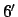, and a maximum error of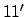.

The ecliptic latitude of the moon can be calculated with the aid of Table 38. The procedure for using this table is as follows:

1. Determine the fractional Julian day number,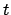, corresponding to the date and time at which the moon's ecliptic latitude is to be calculated with the aid of Tables 27-29. Form, where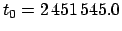is the epoch.
2. Calculate the lunar mean argument of latitude,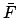, and the five lunar anomalies,-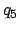, using the procedure outlined earlier in this section.
3. Form the argument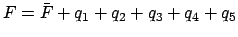. Add as many multiples oftoas is required to make it fall in the rangeto. Roundto the nearest degree.
4. Enter Table 38 with the value ofand take out the lunar ecliptic latitude,. It is necessary to interpolate ifis odd.

For example, we have already seen that at 00:00 UT on May 5, 2005 CE the lunar mean argument of latitude, and the lunar anomalies, were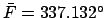, and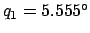,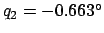,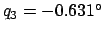,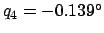, and, respectively. Hence,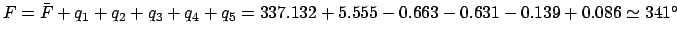. Thus, according to Table 38, the ecliptic latitude of the moon at 00:00 UT on May 5, 2005 CE was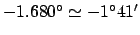.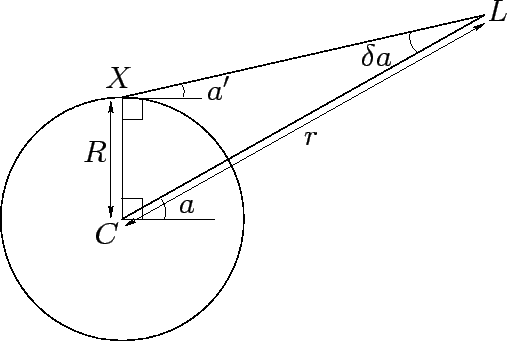Next: Lunar Parallax Up: The Moon Previous: Example Longitude Calculations
Richard Fitzpatrick 2010-07-21